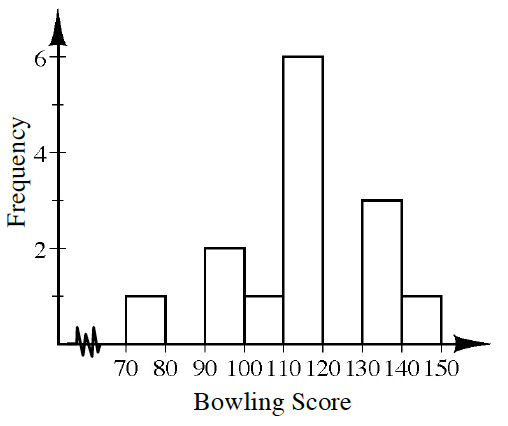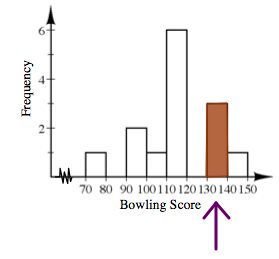### Home > CC1 > Chapter 6 > Lesson 6.1.3 > Problem6-35

6-35.

Audrey made the histogram at right to show her recent bowling scores.

1. How many games did she play in total?

• Each bar height represents the frequency and tells you how many games had a score that fell between the lower and upper value of that bar. For example, $1$ game had a score that fell between $70$ and $79$. Try to add up all the frequencies to find the total number of games.

1. Between what two values did most of her scores fall?

Which bar is the tallest?
Which scores do these bars represent?

Between what score range do at least half of her games fall?
Examine the graph around the tallest bars to see the range where most of the score values fall.

Most of Audrey's scores were between $90$ and $119$.

2. Challenge: What portion of her scores fell between $130$ and $140$?• Examine the bar highlighted in the graph below representing the games played with scores between $130$ and $139$.
How many games were played in this interval?$3$ out of the total $14$ scores fall between $130$ and $139$.

$\text{This can be written as } \frac {3}{14}.$